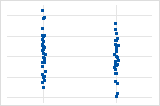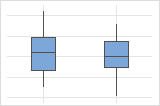# Select the graphs for Test for Equal Variances

Stat > ANOVA > Test for Equal Variances > Graphs
Summary plot
The summary plot includes the multiple comparison intervals for each group or factor level, the standard deviations, and the p-values for the tests.
Individual value plot

Use an individual value plot to assess and compare sample data distributions. An individual value plot works best when the sample size is less than 50. Like a boxplot, an individual value plot helps you to identify potential outliers and see distribution shape. However, unlike a boxplot, an individual value plot displays each value separately. Separate values are especially useful when you have relatively few observations or when assessing the effect of each observation is important.Boxplot

Use a boxplot to assess and compare the shape, central tendency, and variability of sample distributions and to look for outliers. A boxplot works best when the sample size is at least 20. If the sample size is less than 20, consider using an individual value plot instead.By using this site you agree to the use of cookies for analytics and personalized content.  Read our policy